Reach Us+32-10-28-02-25
Solitary Waves for the Modified Korteweg-De Vries Equation in Deterministic Case and Random Case | OMICS International
Journal of Physical Mathematics
All submissions of the EM system will be redirected to Online Manuscript Submission System. Authors are requested to submit articles directly to Online Manuscript Submission System of respective journal.

# Solitary Waves for the Modified Korteweg-De Vries Equation in Deterministic Case and Random Case

Abdelrahman MAE*

Department of Mathematics, Faculty of Science, Mansoura University, Egypt

*Corresponding Author:
Abdelrahman MAE
Department of Mathematics, Faculty of Science
Mansoura University, Egypt
Tel: +20502242388
E-mail: [email protected]

Received Date: January 25, 2017; Accepted Date: February 27, 2017; Published Date: March 03, 2017

Citation: Abdelrahman MAE (2017) Solitary Waves for the Modified Korteweg-De Vries Equation in Deterministic Case and Random Case. J Phys Math 8: 214. doi: 10.4172/2090-0902.1000214

Copyright: © 2017 Abdelrahman MAE. This is an open-access article distributed under the terms of the Creative Commons Attribution License, which permits unrestricted use, distribution, and reproduction in any medium, provided the original author and source are credited.

Visit for more related articles at Journal of Physical Mathematics

#### Abstract

In this paper, we present a new method, the so called Riccati-Bernoulli Sub-ODE method to construct exact traveling wave solutions of the nonlinear modified Korteweg-de Vries (mKdV) equation and also,we use this method in order to solve the nonlinear random modified Korteweg-de Vries (mKdV) equation. It has been shown that the proposed method is effective tools to in order to solve many mathematical physics problems. The travelling wave solutions of these equations are expressed by hyperbolic functions, trigonometric functions and rational functions. The impression of the random coefficient in our problem is studied, by using some distributions through some cases studies.

#### Keywords

Riccati-Bernoulli Sub-ODE method; mKdV equation; Backlund transformation; Traveling wave solutions; Solitary wave solutions; Random variable

#### Introduction

The analytical solution of the linear or nonlinear partial differential equations are so interesting. For this reason, recently, many different methods for finding exact solutions to nonlinear evolution equations have been proposed, developed and extended, such as the Jacobi elliptic function method [1-3], tanh-sech method [4-6], exp-function method [7-9], sine-cosine method [10-12], homogeneous balance method [13,14], F-expansion method [15-17], extended tanh-method [18-21], trigonometric function series method ,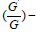expansion method [23-26]. In this article, we introduced the Riccati-Bernoulli sub-ODE technique, to find exact solutions, solitary wave solutions, of nonlinear partial differential equations. By using our technique and a traveling wave transformation, these equations can be converted into a set of algebraic equations. Solving these algebraic equations we get the exact solutions. For illustrating our method we can discuss the modified Kortewegde Vries (mKdV) equation as test case.

The modified Kortewegâ€“de Vries (mKdV) equation is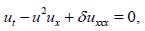(1)

Where δ is a nonzero constant. The expression ut characterize the time evolution of propagating of the wave in one direction. This equation incorporates also two adversary effects: nonlinearity expression as u2ux that accounts in order to seep the wave, and linear dispersion have the form uxx that presented for the spreading of the wave. Nonlinearity leads to localize the wave while propagation prevalences it out, .

The mKdV model describes nonlinear wave propagation in systems with polarity symmetry. The modified Kortewegde Vries (mKdV) equation arises in many applications such as electric circuits and multicomponent plasmas, electrodynamics [26-31].

There are many models have been investigated in the recent decades in physics, chemistry, engineering, and others are formulated as random differential equations (RDEâ€™s). In many situations, models with random inputs are better convenient in describing the actual behaviour for the components in the model than deterministic case [32-34]. Additionally, this work try to study the Riccat-Bernoulli Sub-ODE technique for solving the Modified Korteweg-de Vries Equation (1.1) where δ is a bounded positive random variable. So the spreading coefficient of the wave is a random variable. Some papers have been studied this equation in random case by adding the noise term [35-37], but here we deal with random variable input. If we get a solution of such equations, we can get an infinite solutions sequences of these equations, using a BÃ¤cklund transformation.

The rest of the paper is given as follows: The Riccati-Bernoulli sub- ODE technique is described in Section 4.2.2. In Section 3, a BÃ¤cklund transformation is given. In Section 4 the Riccati-Bernoulli sub-ODE technique is applied to solve the mKdV equation. Finally, Section 5 is devoted to conclusions.

#### The Riccati-Bernoulli sub-ODE technique

Here we give the description of the Riccati-Bernoulli sub-ODE method. Consider the nonlinear evolution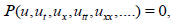(2)

where P is a polynomial in u(x,t) and its partial derivatives with nonlinear terms are embroiled. Now, we give the main steps of this technique :

First step : We use the wave transformation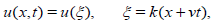(3)

where the wave solution u(ξ) propagate with velocity v and c is a positive constant. Using equation (3), one can transform equation (35) into the following ODE: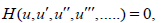(4)

Where H is a polynomial in u(ξ) and its total derivatives, while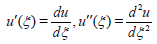and so on.

Second step : Let equation (4) has the formal solution in the following form: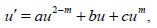(5)

a, b,c and m are constants calculated later. From equation (5), we easily get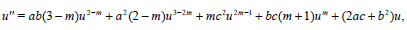(6)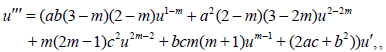(7)

Remark 2.1 When ac≠ 0 and m=0 equation (5) is a Riccati equation. When ac≠ 0 c=0 and m≠ 0 equation (5) is a Bernoulli equation. Both equations are special cases of equation (5). Because equation (5) is firstly introduced, we call equation (5) the Riccati-Bernoulli equation.

Classification Riccati-Bernoulli equation solutions

In fact one can easily check the following cases of solutions for the Riccati-Bernoulli equation (5).

1. A m=1, equation (5) has the solution: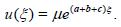(8)

2. At m≠ 1, b=0 and c=0 equation (5) has the solution: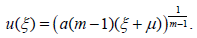(9)

3. At m≠ 1, b≠0 and, c=0 equation (5) has the solution: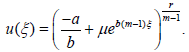(10)

4. At m≠ 1, a≠0 and b2-4ac>0, equation (5) has the solution: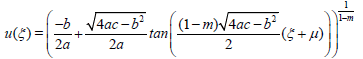(11)

and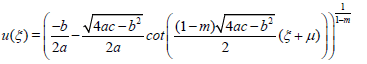(12)

5. At m≠ 1, a≠0 and b2-4ac>0, equation (5) has the solution: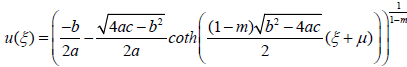(13)

and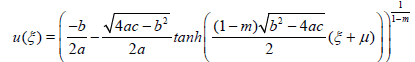(14)

6. At m≠ 1, a≠0 and b2-4ac=0, equation (5) has the solution: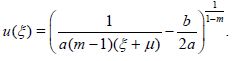(15)

Where μ is an arbitrary constant.

Third step : Setting derivatives of u into equation (4) gives an algebraic equation of u. Note that the symmetry of the right-hand item of equation(5) the highest power exponents of u to equivalence in equation (4), m can be determined. If we compare the coefficients of ui, then we have a set of algebraic equations for a,b,c, and v Solving these equations with substituting m, a,b,c, and v and ξ = k(x + vt) into equations (8)-(15)), we solve equation (35).

#### Bäcklund Transformation

When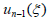and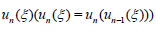are the solutions of equation (5), we get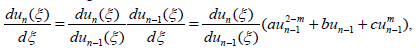namely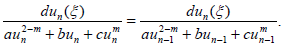(16)

Integrating equation (16) with respect to ξ and after a straight but tedious computation, we get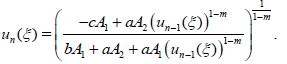(17)

Where A1 and A2 are constants.

Equation (17) is a Bäcklund transformation of equation (5). By getting a solution of this equation, we can construct infinite sequence of solutions of equation (5) by using equation (17).

#### Application

Here, we apply Riccat-Bernoulli Sub-ODE technique to solve the mKdV model , in the deterministic case and random case as we will discuss.

#### The mKdV in Deterministic Case

Recall the modified Korteweg-de Vries (mKdV) equation: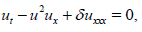(18)

where δ is a nonzero constant. Using the transformation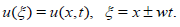(19)

Equation (18) transforms into the following ODEs, using (19):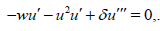(20)

By integrating equation (20) with respect to ξ once, we have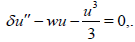(21)

Substituting equations (6) into equation (21), we obtain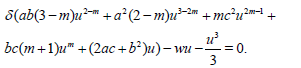(22)

Setting m=0 equation (22) is reduced to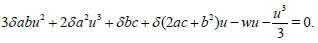(23)

Taking each coefficient of ui(i=0,1,2,3) to zero, we get

δbc = 0, (24)

δ(2ac + b2) − w = 0, (25)

3δ ab = 0, (26)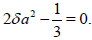(27)

Solving equations (24)-(27), we get

b = 0, (28)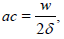(29)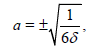(30)

Case I. When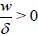, substituting equations (28)-(30) and (19) into equations (11) and (12), we obtain the exact solutions of equation (18) as follow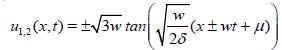(31)

and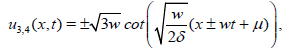(32)

Where w and δ are arbitrary constants. The solution u1 is depicted in Figure 1.

Figure 1: Solution u = u1 (x,t) of the mKdV equation with w=2, δ=0.4 and −4 ≤ t, x ≤ 4 .

Case II. When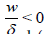, substituting equations (28)-(30) and (19) into equations (13) and (14), the exact solutions of equation (18) is obtained as follow,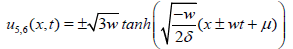(33)

and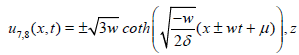(34)

where w and δ are arbitrary constants. The solution u5 is depicted in Figure 2.

Figure 2: Solution u = u5 (x,t) of the mKdV equation with w =2, δ=-0.4 and −4 ≤ t, x ≤ 4 .

Remark 4.1 Applying equation (17) to ui(x,t) (i =1,2,…,8) once, we get an infinite sequence of solutions of equation (18).

The mKdV equation in random case

As we study this method in deterministic case we can development the random case. Since,If we use this method for solving any random differential equation then, the Riccati-Bernoulli sub-ODE solutions are be random variables so, In this section we discuss the stability and convergence theorems for that method.

Theory of stability and convergence for Random Riccati-Bernoulli sub-ODE method: The description of the random Riccati- Bernoulli sub-ODE method. Consider the following nonlinear random evolution equation,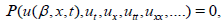(35)

where P is a polynomial in u(β,x,t) and its partial derivatives such that the highest order derivatives as well as nonlinear terms are also involved, is a random variable input as a coefficient. Now, we give the main stability theorem for our method as follow:

Theorem 4.2 The Random Riccati-Bernoulli sub-ODE method is stable and convergent under the conditions:

(1)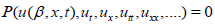is a function of a bounded random variable β, such that: β1<β<β2; β1, β2 are positive constants.

(2)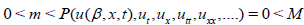; m,M are positive constants.

(3)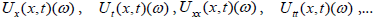are uniformly random bounded.

By these assumptions we can get convergent solutions and also stable for the Random Riccati-Bernoulli sub-ODE method

Mathematical model: Here we develop the theory of the Riccat- Bernoulli Sub-ODE technique to the mKdV equation in random case as in the form: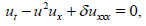(36)

where δ is a random variable. By applying our technique the Riccat- Bernoulli Sub-ODE method to the random equation (36) but when δ is a bounded positive random variable i.e., 0 <δ ≤δ1 where δ1>0, we can make the same steps like as in the deterministic case until we find the solutions for our problem (36) as follows,

When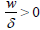, substituting equations (28)-(30) and (19) into equations (11) and (12), we can find the random travelling wave solutions of equation (36) as follow,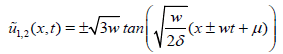(37)

and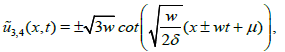(38)

where δ is a random variable and w is arbitrary constant. The expectation value of solutions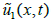and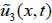are depicted in Figures 3 and 4 for some random distributions illustated in Figures 3 and 4.

Figure 3: Solution u = u1 (x,t) of the mKdV equation with w=2, δ=0.4 and −4 ≤ t, x ≤ 4 .

Figure 4: Graph of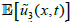of the nonlinear random mKdV equation for w =1, μ=1, δ has Beta and Binomial distributions and −2 ≤ t, x ≤ 2 , where, E[ ] denotes the expectation value operator.

#### Conclusion

In this work, the new method, Riccati-Bernolli Sub-ODE is introduced to find the exact traveling wave solutions of the modified Korteweg-de Vries (mKdV) problem in deterministic and random cases. Expectation value of the exact random process have been shown through some random distributions.

#### References

Select your language of interest to view the total content in your interested language

### Article Usage

• Total views: 1912
• [From(publication date):
March-2017 - Nov 14, 2019]
• Breakdown by view type
• HTML page views : 1745Can't read the image? click here to refresh